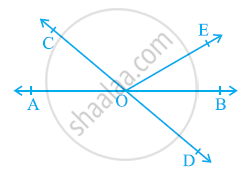# In the given figure, lines AB and CD intersect at O. If ∠AOC + ∠BOE = 70° and ∠BOD = 40°, find ∠BOE and reflex ∠COE. - Mathematics

In the given figure, lines AB and CD intersect at O. If ∠AOC + ∠BOE = 70° and ∠BOD = 40°, find ∠BOE and reflex ∠COE.#### Solution

AB is a straight line, rays OC and OE stand on it.

∴ ∠AOC + ∠COE + ∠BOE = 180°

⇒ (∠AOC + ∠BOE) + ∠COE = 180°

⇒ 70° + ∠COE = 180°

⇒ ∠COE = 180° - 70° = 110°

Reflex ∠COE = 360° - 110° = 250°

CD is a straight line, rays OE and OB stand on it.

∴ ∠COE + ∠BOE + ∠BOD = 180°

⇒ 110° + ∠BOE + 40° = 180°

⇒ ∠BOE = 180° - 150° = 30°

Concept: Pairs of Angles
Is there an error in this question or solution?

#### APPEARS IN

NCERT Class 9 Maths
Chapter 6 Lines and Angles
Exercise 6.1 | Q 1 | Page 96